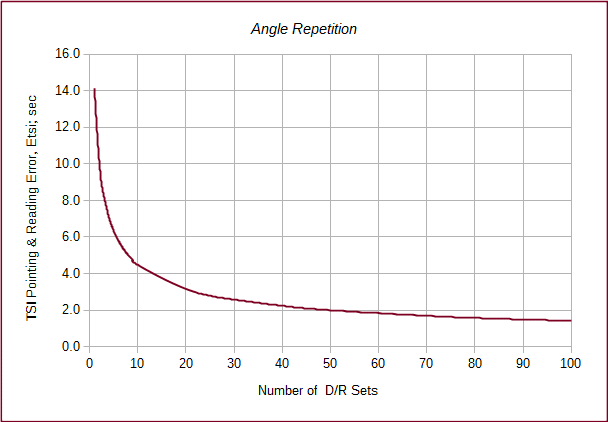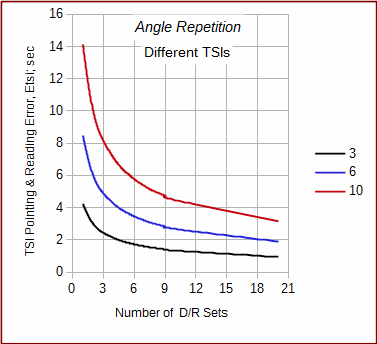## 6. Angle repetition

Measuring the same entity multiple times allows random errors to compensate. Although they can never be entirely eliminated their effect can be lessened through repeated measurements. Measuring an angle direct and reverse is done to compensate for systematic instrumental errors not random errors. The average of a D/R set constitutes a single measurement; it's that resulting angle which has random errors. So to reduce random errors, we repeat D/R sets.

When measuring an angle, once the TSI and targets are set up, their centering error effects do not change during the measurement set. The only thing affecting the final error in the measured angle is the number of times the angle is measured. As seen in the analysis at the end of the second example problem, increasing the repetition from 3 D/R to 4 D/R reduces the expected error from 09.1" to 08.8".

If vary the number of D/R sets in Equation E-3 we can see how it affects the TSI Pointing and Reading error. Figure E-14 shows the effect on TSI pointing and reading error as the number of repetitions is increased. Initially, there is a large accuracy improvement up to about 6 D/R. Once past 20 or so sets, additional measurements yield smaller error improvements.Figure E-14 Angle Repetition Effect

Figure E-15 compares three TSIs with different ISO angle uncertainties while varying D/R sets. At fewer D/R sets there is a larger error difference between the TSIs. As the number of D/R sets increase, the error differences become smaller.Figure E-15 Different TSI Resolutions

Figure E-15 can also be used to determine how many D/R sets are needed to meet a specific Etsi. For example, to achieve a 4" accuracy:

* the 10" TSI would require 13-14 D/R sets
* the 6" TSI would require 4-5 sets
* the 3" TSI would require 1-2 sets

An example of how this is applied is in the FGCS' Standards and Specifications for Geodetic Control Networks which contains a number of formal standards for angle measurement. In particular, the angle measurement standards for Precise Traverse include these two tables:

Table 2 - Instrumentation Requirements
Order First   Second   Second   Third   Third
Class   I II I II

Theodolite, least count

0.2"

1.0"

1.0"

1.0"

1.0"

Table 3 - Field Procedures (partial)
Order First   Second   Second   Third   Third
Class   I II I II
Directions

Number of positions

16

8 or 12†

6 or 8*

4

2

Standard deviation of mean not to exceed

0.4"

0.5"

0.8"

1.2"

2.0"

Rejection limit from the mean.

4"

5"

5"

5"

5"

† 8 if 0.2", 12 if 1.0" resolution.
* 6 if 0.2", 8 if 1.0" resolution.
Theodolite observations for first-order and second-order, class I surveys may be made only at night.

(A position is a single D/R set).

The least count in Table 2 is actually the theodolite reading resolution. Although not the same as the DIN or ISO standard for digital equipment, least count was typically used for the same purposes until the 1980s (the reprint of the Standards was in 1984).

Table 3 contains similar information we divined from Figure E-15 except it includes a bit more detail. It formally identifies the number of D/R sets needed along with some variations based on equipment resolution. As a point of interest, note the night time observation requirement.

Keep in mind that this discussion and Figures E-14 and E-15 address only the TSI Pointing and Reading error. The total expected angle error would also include the TSI Centering and Target Centering errors which are independent of angle repetition.